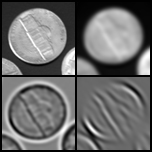# tutor2.jpg

Description:

One MATLAB® tutorial exercise explores how the retinal image (upper left) is processed by neurons in the early stages of the visual pathway. This processing can be modeled as convolution with spatial filters that incorporate Gaussian smoothing (upper right). The result of retinal processing can be described as convolution with the difference of two Gaussians that form a center-surround spatial structure (lower left). The spatial receptive fields of neurons in visual cortex can be described as an oriented Gabor filter, producing results such as that shown for an oblique orientation in the lower right.

Alt text:
Sequence of four images of a coin, with progressively less detail and resolution.
Caption:
One MATLAB® tutorial exercise explores how the retinal image (upper left) is processed by neurons in the early stages of the visual pathway. This processing can be modeled as convolution with spatial filters that incorporate Gaussian smoothing (upper right). The result of retinal processing can be described as convolution with the difference of two Gaussians that form a center-surround spatial structure (lower left). The spatial receptive fields of neurons in visual cortex can be described as an oriented Gabor filter, producing results such as that shown for an oblique orientation in the lower right.#### Learning Resource Types

theaters Other Video
theaters Lecture Videos
notes Lecture Notes
group_work Projects
co_present Instructor Insights